• 使用MATLABplot函数时，想在实现上加实点，刚开始使用的代码如下： figure;plot(rand(1,6),'-.') “-”表示实线，“.”表示实心点，但得到的却是点划线。 找了很久都没有找到答案，一番艰辛后，将代码中的“.
此博客为个人博客，不涉及商业用途，仅提供学习参考，内容均来自个人原创以及互联网转载和摘录。
此博客上带有原创标识的文章、图片、文件等，未经本人允许，不得用于商业用途以及传统媒体。网络媒体或个人转载请注明出处和链接，否则属于侵权行为。
————————————————
在使用MATLAB的plot函数时，想在实现上加实点，刚开始使用的代码如下：
figure;plot(rand(1,6),'-.')

“-”表示实线，“.”表示实心点，但得到的却是点划线。找了很久都没有找到答案，一番艰辛后，将代码中的“.”、“-”换个位置，改成：
figure;plot(rand(1,6),'.-')即可得到实线实点，还可用’MarkerSize’改变点的大小：
figure;plot(rand(1,6),'.-','MarkerSize',14)————————————————
感谢您的阅读，如果您有收获，请给我一个三连吧！
如果您觉得这还不够，可以点击 打赏 按钮，告诉我： 你币有了！


展开全文• 所以今天我们来讲讲这个非常好用的Plot函数 话不多说，上代码 %%获取视频文件 obj = VideoReader('test2_6.avi');%输入视频位置,并获取视频所有帧 numFrames = obj.NumberOfFrames;% 帧的总数   r = []; %分别...
我们在使用matlab进行图像处理的过程中，经常会用到的一个工具就是折线图！不同于直方图，但性质类似！所以今天我们来讲讲这个非常好用的Plot函数

话不多说，上代码

%%获取视频文件
obj = VideoReader('test2_6.avi');%输入视频位置,并获取视频所有帧
numFrames = obj.NumberOfFrames;% 帧的总数

r = []; %分别存放各帧所需的数值

for i = 1 : numFrames %总帧数
frame = read(obj, i);%读取第几帧
frame=double(frame);
image_r=frame(:,:,1);
R=mean(image_r(:)); %计算矩阵的均值
r(i)=R;%将每个图像的均值存入数组中
end

plot(1:numFrames,r,'r');%绘制所有分帧图像矩阵均值折线图
%%RGB曲线图注释说明
axis([1 716 80 130]); %规定x轴和y轴范围
xlabel('帧数');  %曲线图注释说明
ylabel('均值');
title('视频图像均值曲线图');
legend('R');

对于不太了解matlab的朋友，下面我将解释上述代码：

首先，整个视频分为3大部分———1、视频读取；2、利用for循环对视频的每一帧图像进行操作；3、利用plot函数构图

1、视频读取：自己看

2、for循环操作：取决于用户想获取图像中的什么数据，制造什么折线图，这个随意。

但是有一点要注意的-------提前设计好矩阵来存储数据

3、plot函数（重点）：

3.1第一句“plot(1:numFrames,r,'r');%绘制所有分帧图像矩阵均值折线图”，其中“1:numFrames”代表x轴数值，“r”代表y轴数值，

但是注意单引号里面的“r”,代表的是折线的颜色（顺带说一句，折线的颜色和样式可以自行百度，我这里只能列举一些常用的）

标记符    颜色
r          红
g          绿
b          蓝
c          蓝绿
m          紫红
y          黄
k          黑
w          白

3.2坐标轴的样式

%%RGB曲线图注释说明
axis([1 716 80 130]); %规定x轴和y轴范围          “1    716”是指x轴；“80   130”是指y轴

xlabel('帧数');  %曲线图注释说明
ylabel('均值');
title('视频图像均值曲线图');
legend('R');

总体来说，matlab的plot函数并不难，关键的是抓住那几个细节！希望能给需要的朋友带来帮助！！

欢迎大家指正讨论哦！！！


展开全文• 快速入门matlab，系统地整理一遍，如何你和我一样是一个新手，那么此文很适合你；

快速入门matlab，系统地整理一遍，如何你和我一样是一个新手，那么此文很适合你；

文章目录1 前言2 plot2.1 显示正弦波2.2 修改颜色2.3 修改点的形状2.4 修改线的形状2.5 多个参数修改3 subplot3.1 2行1列3.2 1行2列4 plot35 title6 legend7 at last
1 前言
如果你是和我一样的小白，强烈推荐看看这里，需要合理地利用官方的文档，通常我觉得官方文档是最好的，没有之一，在命令终端输入help plot，可以看到详细的帮助文档；具体如下；
>> help plot
plot   Linear plot.
plot(X,Y) plots vector Y versus vector X. If X or Y is a matrix,
then the vector is plotted versus the rows or columns of the matrix,
whichever line up.  If X is a scalar and Y is a vector, disconnected
line objects are created and plotted as discrete points vertically at
X.

plot(Y) plots the columns of Y versus their index.
If Y is complex, plot(Y) is equivalent to plot(real(Y),imag(Y)).
In all other uses of plot, the imaginary part is ignored.

Various line types, plot symbols and colors may be obtained with
plot(X,Y,S) where S is a character string made from one element
from any or all the following 3 columns:

b     blue          .     point              -     solid
g     green         o     circle             :     dotted
r     red           x     x-mark             -.    dashdot
c     cyan          +     plus               --    dashed
m     magenta       *     star             (none)  no line
y     yellow        s     square
k     black         d     diamond
w     white         v     triangle (down)
^     triangle (up)
<     triangle (left)
>     triangle (right)
p     pentagram
h     hexagram

For example, plot(X,Y,'c+:') plots a cyan dotted line with a plus
at each data point; plot(X,Y,'bd') plots blue diamond at each data
point but does not draw any line.

plot(X1,Y1,S1,X2,Y2,S2,X3,Y3,S3,...) combines the plots defined by
the (X,Y,S) triples, where the X's and Y's are vectors or matrices
and the S's are strings.

For example, plot(X,Y,'y-',X,Y,'go') plots the data twice, with a
solid yellow line interpolating green circles at the data points.

The plot command, if no color is specified, makes automatic use of
the colors specified by the axes ColorOrder property.  By default,
plot cycles through the colors in the ColorOrder property.  For
monochrome systems, plot cycles over the axes LineStyleOrder property.

Note that RGB colors in the ColorOrder property may differ from
similarly-named colors in the (X,Y,S) triples.  For example, the
second axes ColorOrder property is medium green with RGB [0 .5 0],
while plot(X,Y,'g') plots a green line with RGB [0 1 0].

If you do not specify a marker type, plot uses no marker.
If you do not specify a line style, plot uses a solid line.

plot(AX,...) plots into the axes with handle AX.

plot returns a column vector of handles to lineseries objects, one
handle per plotted line.

The X,Y pairs, or X,Y,S triples, can be followed by
parameter/value pairs to specify additional properties
of the lines. For example, plot(X,Y,'LineWidth',2,'Color',[.6 0 0])
will create a plot with a dark red line width of 2 points.

Example
x = -pi:pi/10:pi;
y = tan(sin(x)) - sin(tan(x));
plot(x,y,'--rs','LineWidth',2,...
'MarkerEdgeColor','k',...
'MarkerFaceColor','g',...
'MarkerSize',10)



与plot相关的函数还有 plottools, semilogx, semilogy, loglog, plotyy, plot3, grid,title, xlabel, ylabel, axis, axes, hold, legend, subplot, scatter.

2 plot
2.1 显示正弦波
显示一个简单的正弦函数；
x=0:2*pi/100:2*pi;
y=sin(x);
plot(x,y);2.2 修改颜色

参数
颜色

b
blue

g
green

r
red

c
cyan

m
magenta

y
yellow

k
black

w
white

下面修改为红色：
x=0:2*pi/100:2*pi;
y=sin(x);
plot(x,y,'r');

结果如下：2.3 修改点的形状

参数
形状
图标

-
solid

o
circle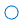x
x-mark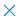+
plus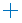*
star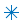s
square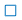d
diamond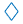v
triangle (down)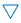^
triangle (up)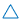<
triangle (left)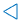>
triangle (right)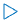p
pentagram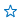h
hexagram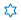将点形状显示为六边形；
x=0:2*pi/20:2*pi;
y=sin(x);
plot(x,y,'h','MarkerSize',10);

结果如下：相关参数：

MarkerEdgeColor：点边框颜色；
MarkerFaceColor：点表面颜色；
MarkerSize：点的大小；

2.4 修改线的形状

符号
形状

:
dotted

-.
dashdot

--
dashed

x=0:2*pi/20:2*pi;
y=sin(x);
plot(x,y,':','LineWidth',3);

LineWidth的参数为线宽；x=0:2*pi/20:2*pi;
y=sin(x);
plot(x,y,'-.','LineWidth',3);x=0:2*pi/20:2*pi;
y=sin(x);
plot(x,y,'--','LineWidth',3);2.5 多个参数修改
下面修改多个参数属性显示一下正弦波；
x = 0:2*pi/100:2*pi;
y = sin(x);
plot(x,y,'--rs','LineWidth',2,...
'MarkerEdgeColor','k',...
'MarkerFaceColor','g',...
'MarkerSize',10);

结果如下：3 subplot
subplot的使用方法如下：
 subplot Create axes in tiled positions.
H = subplot(m,n,p), or subplot(mnp), breaks the Figure window
into an m-by-n matrix of small axes, selects the p-th axes for
the current plot, and returns the axes handle.  The axes are
counted along the top row of the Figure window, then the second
row, etc.  For example,

subplot(2,1,1), PLOT(income)
subplot(2,1,2), PLOT(outgo)

通俗的讲：
subplot(行，列，index)
注意：plot函数要在subplot表明位置之后再调用。
3.1 2行1列
x=0:2*pi/20:2*pi;
y=sin(x);
subplot(2,1,1);
plot(x,y,'y','LineWidth',3);
subplot(2,1,2);
plot(x,y,'g','LineWidth',3);

需要将多个波形显示在同一张图中；3.2 1行2列
x=0:2*pi/20:2*pi;
y=sin(x);
subplot(1,2,1);
plot(x,y,'y','LineWidth',3);
subplot(1,2,2);
plot(x,y,'g','LineWidth',3);4 plot3
 t = 0:pi/50:10*pi;
plot3(sin(t),cos(t),t);5 title

title：图的标题；
xlabel：x轴标题；
ylabel：y轴标题；
指定plot的标题，需要在plot调用之后在调用title，xlabel或ylabel；

x=0:2*pi/20:2*pi;
y=sin(x);

subplot(1,2,1);

plot(x,y,'y','LineWidth',3);
title('yellow');
xlabel('yellow-x');
ylabel('yellow-y');
subplot(1,2,2);
plot(x,y,'g','LineWidth',3);
title('green');
xlabel('green-x');
ylabel('green-y');6 legend
x = 0:.2:12;
plot(x,besselj(1,x),x,besselj(2,x),x,besselj(3,x));
legend('First','Second','Third','Location','NorthEastOutside')

b = bar(rand(10,5),'stacked'); colormap(summer); hold on
x = plot(1:10,5*rand(10,1),'marker','square','markersize',12,...
'markeredgecolor','y','markerfacecolor',[.6 0 .6],...
'linestyle','-','color','r','linewidth',2); hold off
legend([b,x],'Carrots','Peas','Peppers','Green Beans',...
'Cucumbers','Eggplant')这是官方的demo，比较复杂；
x=0:2*pi/20:2*pi;
y=sin(x);

plot(x,y,':','LineWidth',3);
legend('test1');legend需要在plot之后调用，用于依次解释第一个plot的波形，如果一个plot里显示了两个波形，那legen中字符串也需要设置两个，分别依次对应plot中的波形；
7 at last
比较简单，matplotlib的功能和这个比较类似，总体来说，作为一个和博主一样的新手，要多看官方的help文档，然后平时使用的过程中慢慢就熟悉了，最后要多总结。

作者能力有限，文中难免有错误和纰漏之处，请大佬们不吝赐教
创作不易，如果本文帮到了您；
请帮忙点个赞 👍👍👍；
请帮忙点个赞 👍👍👍；
请帮忙点个赞 👍👍👍；


展开全文• 文末有示例文件获取方法工作中，我们经常需要找出包含指定内容的信息，比如...出现指定字符自动标注颜色标注过程自动标注的核心在于指定内容的识别，这里是使用了COUNTIF函数来识别姓名中是否包含特定字符，标注过程...
文末有示例文件获取方法工作中，我们经常需要找出包含指定内容的信息，比如：某个姓氏的员工，某种产品的品名，某个公司的简称等等，使用筛选需要进行大量重复操作，有没有什么方法，可以自动标出所有信息呢？今天，技巧君就来分享一下，如何让Excel出现指定字符时，自动标注颜色！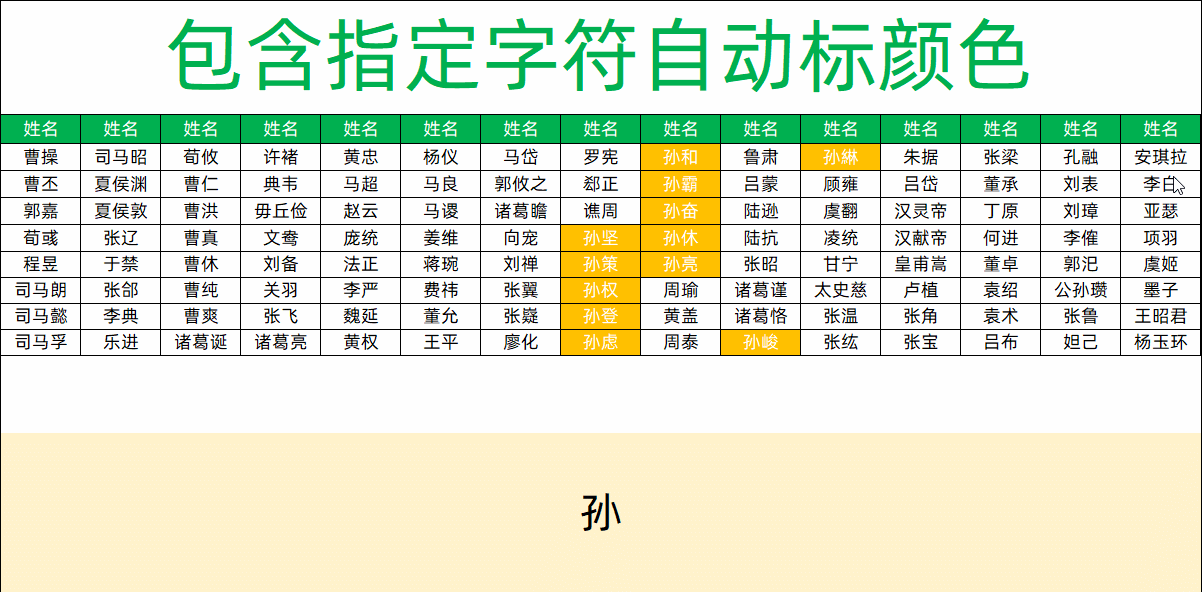出现指定字符自动标注颜色标注过程自动标注的核心在于指定内容的识别，这里是使用了COUNTIF函数来识别姓名中是否包含特定字符，标注过程主要有两步：使用COUNTIF函数识别出包含特定字符的姓名设置条件格式，实现颜色自动标注COUNTIF函数在开始标注之前，我们先来认识一下COUNTIF函数：COUNTIF函数功能：按照指定条件进行计数语法：COUNTIF(计数范围，计数条件)示例：=COUNTIF(A2:A8,E1)，在A2-A8中，计算“冰箱”出现了几次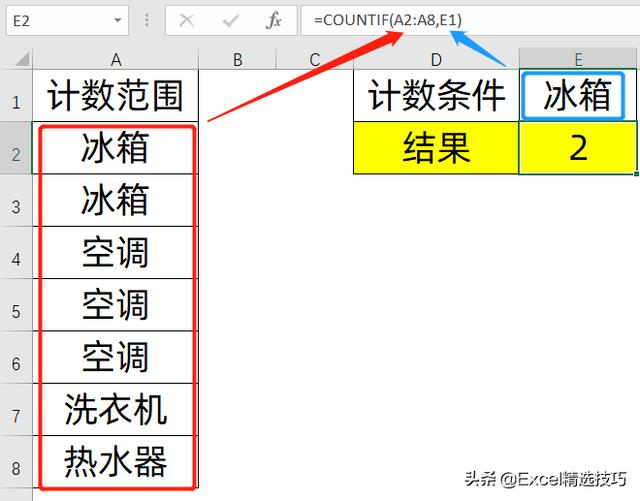COUNTIF函数示例用COUNTIF函数识别指定内容我们利用COUNTIF函数来标注每个姓名，是否包含指定的字符，如果包含，会得到 1 ，如果不包含，则得到 0，公式为：=COUNTIF(A3,$O$1&"*")表示在姓名中，以“孙”开头的文本有几个“*”是Excel中的通配符，匹配任意字符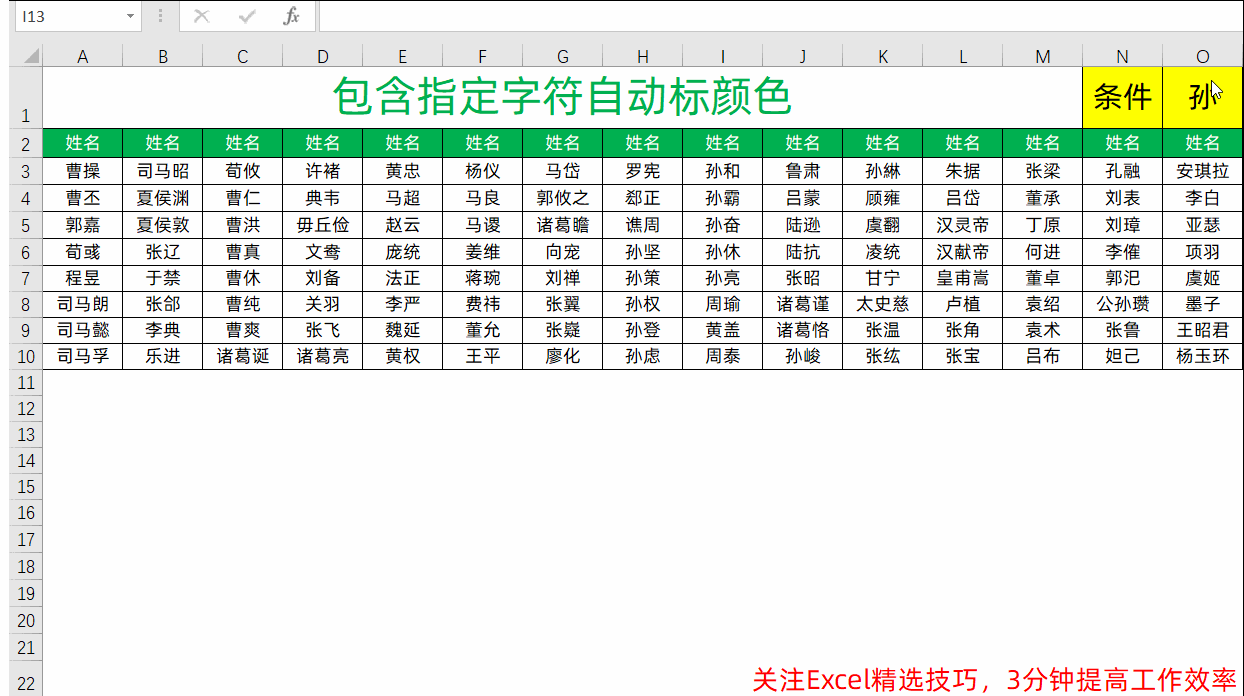用COUNTIF识别指定内容设置条件格式使用上面的公式，设置条件规则，并按照需要设置好格式选中姓名区域→条件格式→新建规则→使用公式确定要设置格式的单元格→=COUNTIF(A3,$O$1&"*")→设置要标注的颜色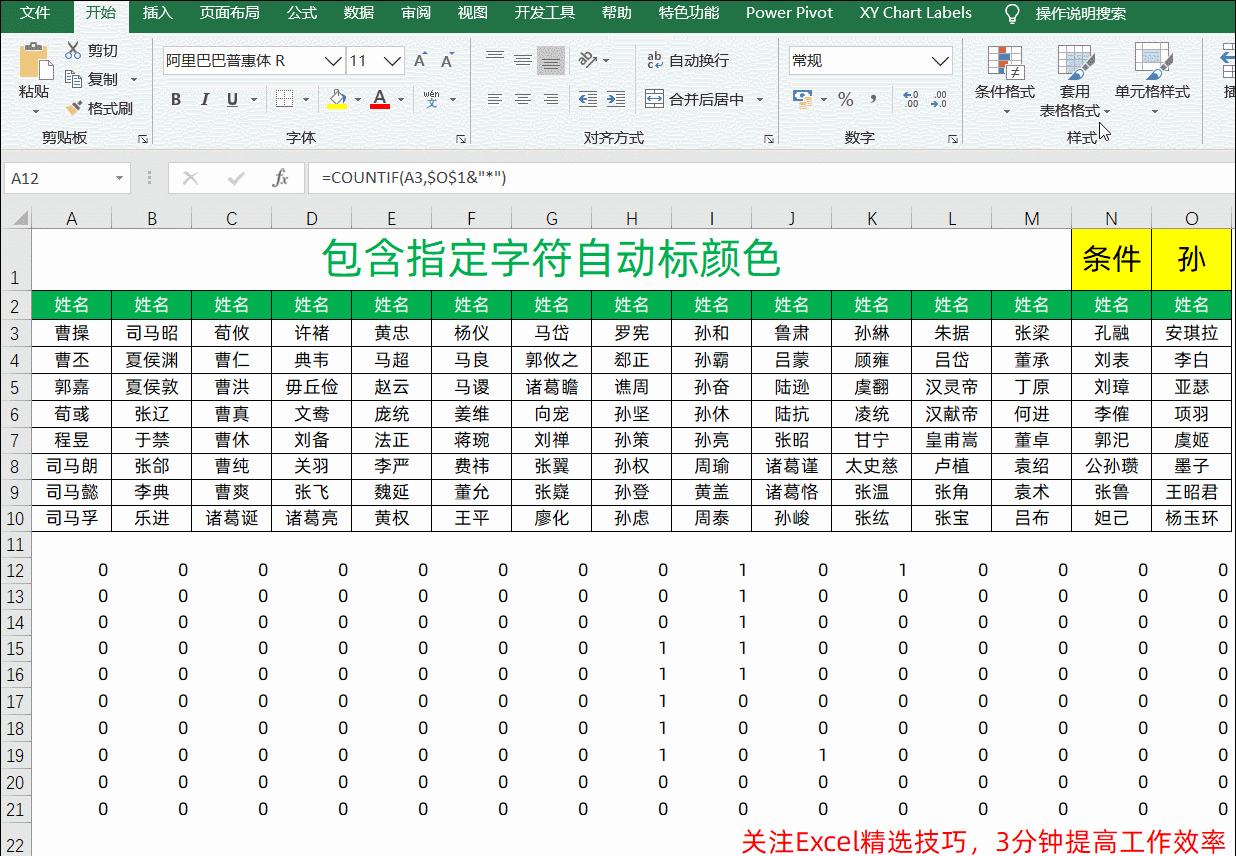设置条件格式示例文件获取方法关注我之后，私信发送【20200409】，即可获取！记得点赞、收藏、转发哦！
展开全文• 快速入门matlab，系统地整理一遍，如何你和我一样是一个新手，那么此文很适合你；1 前言如果你是和我一样的小白，强烈推荐看看这里，需要合理地利用官方的文档，通常我觉得官方文档是最好的，没有之一，在命令终端...
• 我们在matlab中经常会用到plot函数做出数学曲线，其实matplotlib就是模仿matlab这样风格作图的，它是由Hunter教授开发的一开始用于科研图表制作的一个库。下面我将以散点图为例，示范如何绘制一幅完整的matplot图像...
• !... 如图，显示了三幅图，为便于比较，希望能让三幅图以相同纵坐标范围来...平常的操作是先画出来，观察哪一个纵坐标范围最大，然后对每张图分别使用ylim函数.想知道有没有什么方法能够通过代码获各图的纵坐标范围？百度云
• plot制图是MATLAB最为简单也最为常用的制图函数，其工作原理为将两个一维数组一一对应后在坐标系上描点作图。本文将简要介绍如何使用plot函数以及其相关功能制作出一个符合自己要求的图窗。
• 使用高斯函数作为测试用例不是很恰当，计算出来的半高宽都一样。我在peaks函数的基础上改出来一个多峰函数，还存在一些小问题，但基本上可用了，你可以先看看：X=-10:.5:10;Y=-10:.5:10;FWHM=X*0;fori=1:length(X)x=...
• 本示例说明如何使用函数在左侧和右侧创建带有y-轴的图表yyaxis。它还显示了如何标记每个轴，组合多个图以及清除与一侧或两侧有关的图。相对于左y轴绘制数据创建左侧和右侧为y轴的轴。该yyaxis left命令创建轴并...
• MATLA中如何求分段函数的图像 比如我们有如下分段函数 方法一、使用综合表达式 clear;clf; x=-10:0.1:10; y=x.2+(10+x).(1/4)+3x.(x>0)+0.(x==0)+x.^3+sqrt(10-x)-3x.(x<0); plot(x,y,‘r–’,‘LineWidth’,...
• 本文阐述如何使用Matlab来实现这些功能。 1、如何使用Matlab绘制相关曲线图 在Matlab中，常采用plot函数来绘制二维图像。其函数格式如下： plot(x,y) 如果x,y都属于向量，则它们必须具有相同的长度，plot函数将以...
• 这里写自定义目录标题欢迎使用Markdown编辑器新的改变功能快捷键合理的创建标题，有助于目录的生成如何改变文本的样式插入链接与图片如何插入一段漂亮的代码片生成一个适合你的列表创建一个表格设定内容居中、居左、...
• 目标图片如下： ...plot(x,y1,’–go’,x,y2,’:r*’,x,y3,’-.bs’)%画图同时确定线形、颜色和点的形状； legend(‘y = sin(x/3)’,‘y2 = sin(x-pi/4)’,‘y3=sin(x)’)%生成注解 title(‘Graph of
• 本文讲述如何使用python3分析模拟电子线路中的波特图问题，以及如何使用类似Matlab函数进行绘图python3 科学计算
• MATLAB绘图处理实用函数欢迎使用Markdown编辑器新的改变功能快捷键合理的创建标题，有助于目录的生成如何改变文本的样式插入链接与图片如何插入一段漂亮的代码片生成一个适合你的列表创建一个表格设定内容居中、居左...数据可视化 程序设计
• ## MATLAB中figure的使用

千次阅读 2018-09-22 23:36:01
当我们调用plot( )函数时,MATLAB会自动生成一个命名为Figure1的窗体，那么我们如何修改它的名字呢。 教程如下： figure('NumberTitle', 'off', 'Name', 'CSJ测试'); MATLAB中subplot函数使用 subplot(1,2,1)...figure
• 本示例说明如何通过更新三角形的数据属性来围绕圆内部循环动画x显示三角形。绘制圆并设置轴边界，... axis equal使用area函数绘制平面三角形。然后，使用圆的（x，y）坐标更改三角形顶点之一的值。循环更改值以创建...
• MATLAB中绘制条形图的方法比较简单，给定样本值，直接调用bar()函数即可完成基本的条形图的绘制，bar3()可以...或者与plot(x,y)函数方法相同，先定义x, 如x=0:pi/10:pi，再使用bar(x,y)。其他关于bar()函数的属性不常用条形图
• Matlab使用Plot函数实现数据动态显示方法总结中介绍了两种实现即时数据动态显示的方法。考虑到使用python的人群日益增多，再加上本人最近想使用python动态显示即时的数据，网上方法很少，固总结于此。示例代码1...
• Matlab使用Plot函数实现数据动态显示方法总结中介绍了两种实现即时数据动态显示的方法。考虑到使用python的人群日益增多，再加上本人最近想使用python动态显示即时的数据，网上方法很少，固总结于此。示例代码1...
• plot（）函数之会保存最近所执行的数据，如果连续使用两次plot则只会保存第二次的图像 hold on/off函数 如果想连续画多个图像则需要 hold on; plot(cos(0:pi/20:2*pi)) ; plot(sin(0:pi/20:2*pi)) ;...
• 如何用符号函数来做图 最近在学习使用符号函数，在此过程中遇到了许多问题，尤其是我不太清楚应该用什么函数来画...首先，要知道plot函数是画数值图像的，而函数、函数句柄根本就没有具体数值，人家还等着你去赋值...编程语言 数学建模
• %设一被控对象G（s）=50/(0.125s^2+7s), %用增量式PID控制算法编写仿真程序 %（输入分别为单位阶跃、正弦信号，采样时间为1ms，控制器输出限幅：[-5,5], ... 该如何使用tfdata并修改公式计算yout？开发语言 人工智能 自动驾驶 git
• 但是我们使用plot函数绘图时候，加了分号也没用，还是会直接绘图出来了 当我们按下回车键，发现&lt;&lt;这个出来了，说明直接打印出当前能打印的（没有加；分号抑制的）变量 而按下shift+enter键可以实现...
• Matlab改变绘图的坐标轴网格精度； 怎么用matlab画圆； matlab如何定义函数； matlabplot函数表示颜色； matlab画图怎么设置线条类型和颜色； 使用matlab来绘制voronoi图。function
• Matlab提供了一系列绘图函数，常见的包括绘制2D曲线的plot函数、绘制2D隐函数曲线的ezplot函数、绘制3D曲面的mesh和surf函数、绘制3D显函数曲面的ezmesh和ezsurf函数。值得注意的是，ez系列的绘图函数里只有ezplot是...
• 上一讲介绍了二维的画图以及一个函数 plot() 的详细介绍，今天看matlab中三维绘图。 今天我们会学会如何matlab中绘制简单的三维图像，以及理解一些常用函数。 开始啦 三维中有plot()吗？ 在三维画图中，有对应...可视化
• 本示例说明如何在阶梯图上叠加线图。定义要绘制的数据。 alpha = 0.01; beta = 0.5; t = 0:10; f = exp(-alpha*t).*sin(beta*t);显示f为阶梯图。使用hold功能可以保留阶梯图。使用数据f，添加带有星形标记的虚线...
• 1.绘制二维曲线的最基本函数plot2.双纵坐标函数plotyy3.坐标控制函数的调用格式为：axis([xmin xmax ymin ymax zmin zmax])axis函数功能丰富，常用的用法还有：axis equal 纵、横坐标轴采用等长刻度axis square 产生...# matlabplot函数如何使用matlab 订阅